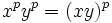Frattini-in-center odd-order p-group implies p-power map is endomorphism

This article states and (possibly) proves a fact that is true for odd-order p-groups: groups of prime power order where the underlying prime is odd. The statement is false, in general, for groups whose order is a power of two.
View other such facts for p-groups|View other such facts for finite groups

Statement

Suppose$p$ is an odd prime, and$P$ is a finite$p$-group (i.e., a group of prime power order) that is a Frattini-in-center group: the Frattini subgroup of$P$ is contained in its center. Then, the map$x \mapsto x^p$ is an endomorphism of$P$.

Note that this makes it a universal power endomorphism, i.e., an endomorphism described everywhere as raising to a certain power. The endomorphism is nontrivial only if$P$ does not itself have exponent$p$.

Examples

The smallest non-abelian examples for any odd prime$p$ are the two non-abelian groups of order$p^3$, namely unitriangular matrix group:UT(3,p) (GAP ID$(p^3,3)$) and semidirect product of cyclic group of prime-square order and cyclic group of prime order (GAP ID$(p^3,4)$). Of these two groups, the former has exponent$p$, so the$p$-power map is the trivial endomorphism. The latter has exponent$p^2$, so the$p$-power map is a nontrivial endomorphism.

In the case$p = 3$, these groups are unitriangular matrix group:UT(3,3) and semidirect product of Z9 and Z3 respectively. Both groups have order$3^3 = 27$.

Proof

This proof uses a tabular format for presentation. Provide feedback on tabular proof formats in a survey (opens in new window/tab) | Learn more about tabular proof formats|View all pages on facts with proofs in tabular format

Given: An odd prime$p$. A finite$p$-group$P$, such that$P/Z(P)$ is elementary Abelian.

To prove: The map$x \mapsto x^p$ is an endomorphism of$P$. Specifically$(xy)^p = x^py^p$ for any$x,y \in P$.

Proof:

Step no. Assertion Given data used Facts used Previous steps used Explanation
1 The derived subgroup$[P,P]$ is elementary abelian. In particular,$[x,y]^p$ is the identity element for any$x,y \in P$.$P$ is Frattini-in-center Fact (1) -- Follows directly from fact (1).
2$p$ divides$p(p-1)/2$.$p$ is an odd prime. -- -- Basic properties of divisibility. Note that this breaks down for$p = 2$, because of the$2$ in the denominator.
3$[x,y]^{p(p-1)/2}$ is the identity element for all$x,y \in P$. Steps (1), (2) By step (1),$[x,y]^p$ is the identity element, so the order of$[x,y]$ divides$p$. Since$p$ divides$p(p-1)/2$, the order of$[x,y]$ divides$p(p-1)/2$, so$[x,y]^{p(p-1)/2}$ is the identity element.
4 We have the formula$x^py^p = [x,y]^{p(p-1)/2}(xy)^p$ for all$x,y \in P$.$P$ is Frattini-in-center, and hence class two. Fact (2) -- Because$P$ is Frattini-in-center, the quotient by the center is elementary abelian, and hence abelian, so$P$ has class at most two. Thus, we can use fact (2) to get the formula.
5$x^py^p = (xy)^p$ for all$x,y \in P$ Steps (3), (4) This follows directly by plugging in the conclusion of step (3) into step (4).